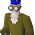## Here is a JAVA Program to find the distance between two GPS Coordinates.

static double latitude1 = 40.6971494;
static double latitude2 = 19.0821978;
static double longitude1 = -74.2598702;
static double longitude2 = 72.7410985;

public static void findDistance()
{

double lon1 = Math.toRadians(longitude1);
double lon2 = Math.toRadians(longitude2);
double  lat1 = Math.toRadians(latitude1);
double  lat2 = Math.toRadians(latitude2);

double dlon = lon2 - lon1;
double dlat = lat2 - lat1;
double a = Math.pow(Math.sin(dlat / 2), 2)
+ Math.cos(lat1) * Math.cos(lat2)
* Math.pow(Math.sin(dlon / 2),2);

double c = 2 * Math.asin(Math.sqrt(a));

// Radius of earth in kilometers. Use 3956
// for miles
double r = 6371;

// calculate the result
double distance = c * r;

System.out.println("Distance in KM : "+ Math.floor(distance));
System.out.println("Distance in Miles : "+ Math.floor(distance*.621371));
System.out.println("Distance in Nautical Miles : "+ Math.floor(distance*.539957));

}

#### 1 comment:

1.In the web based market, there are plenty of systems available where you could buy osrs gold and one of the best websites in this regard is MMOGAH. By trading and exchanging, game enthusiasts are allowed to acquire adequate gold inside their accounts giving mmogah.com/osrs-gold them an excellent expertise in the video game.